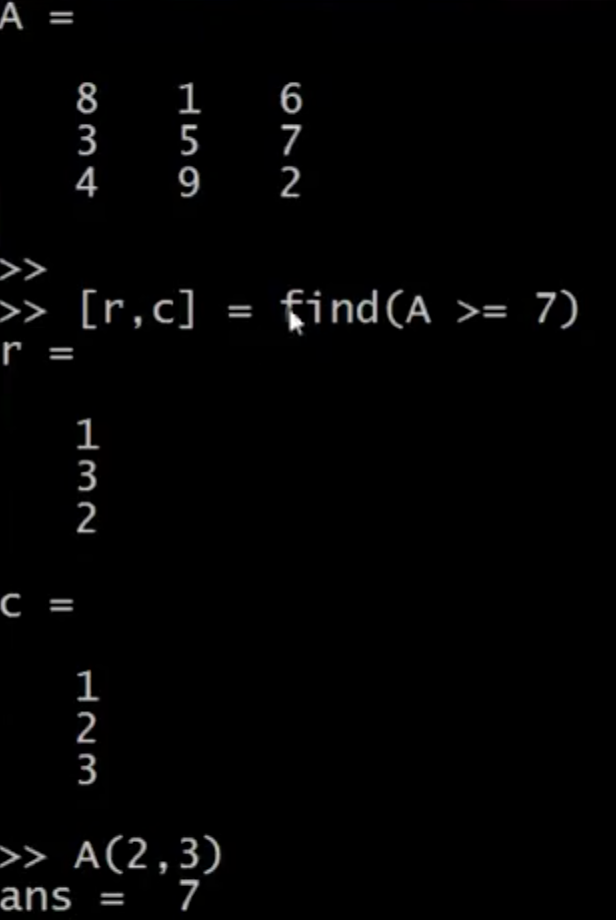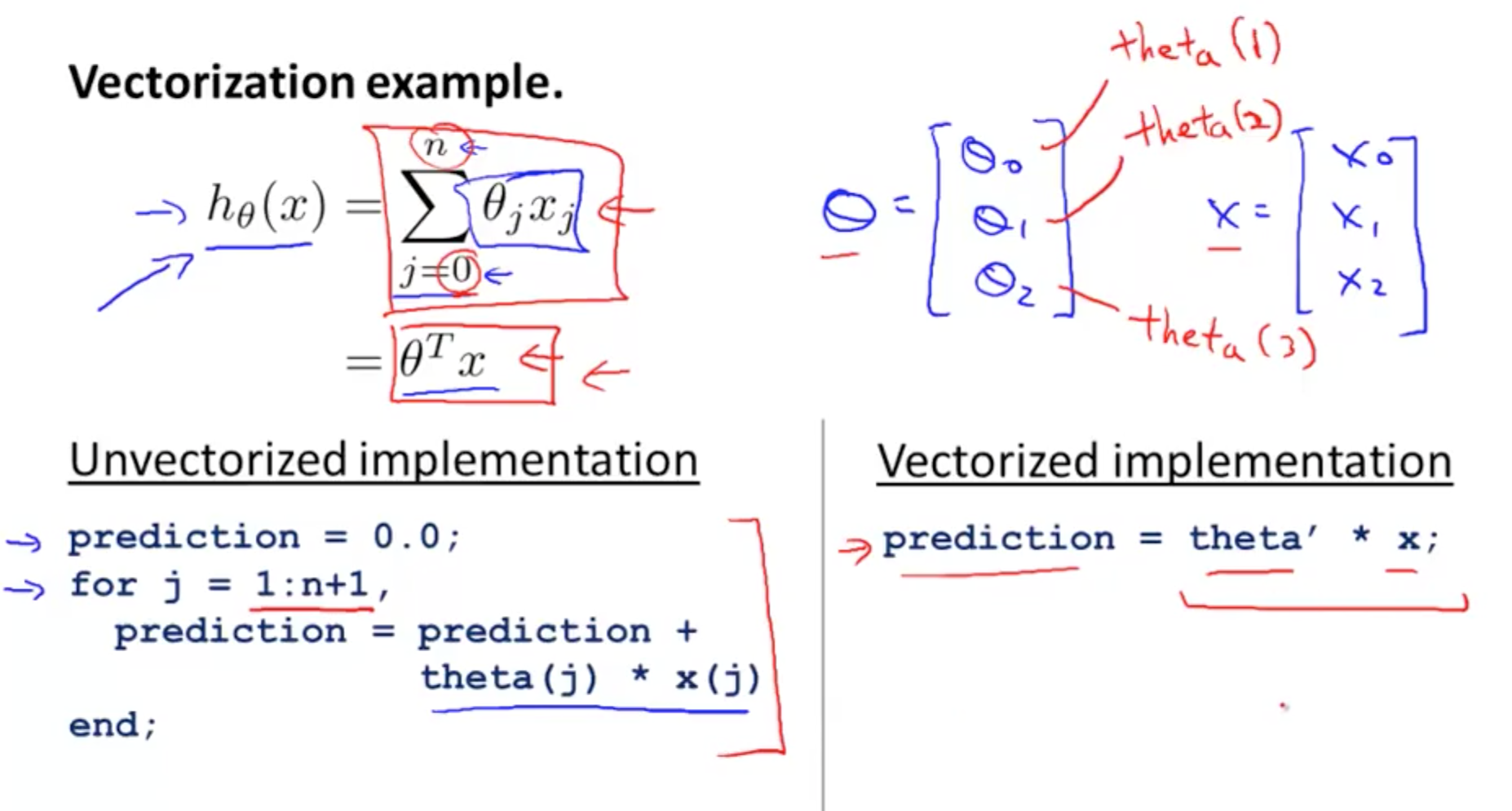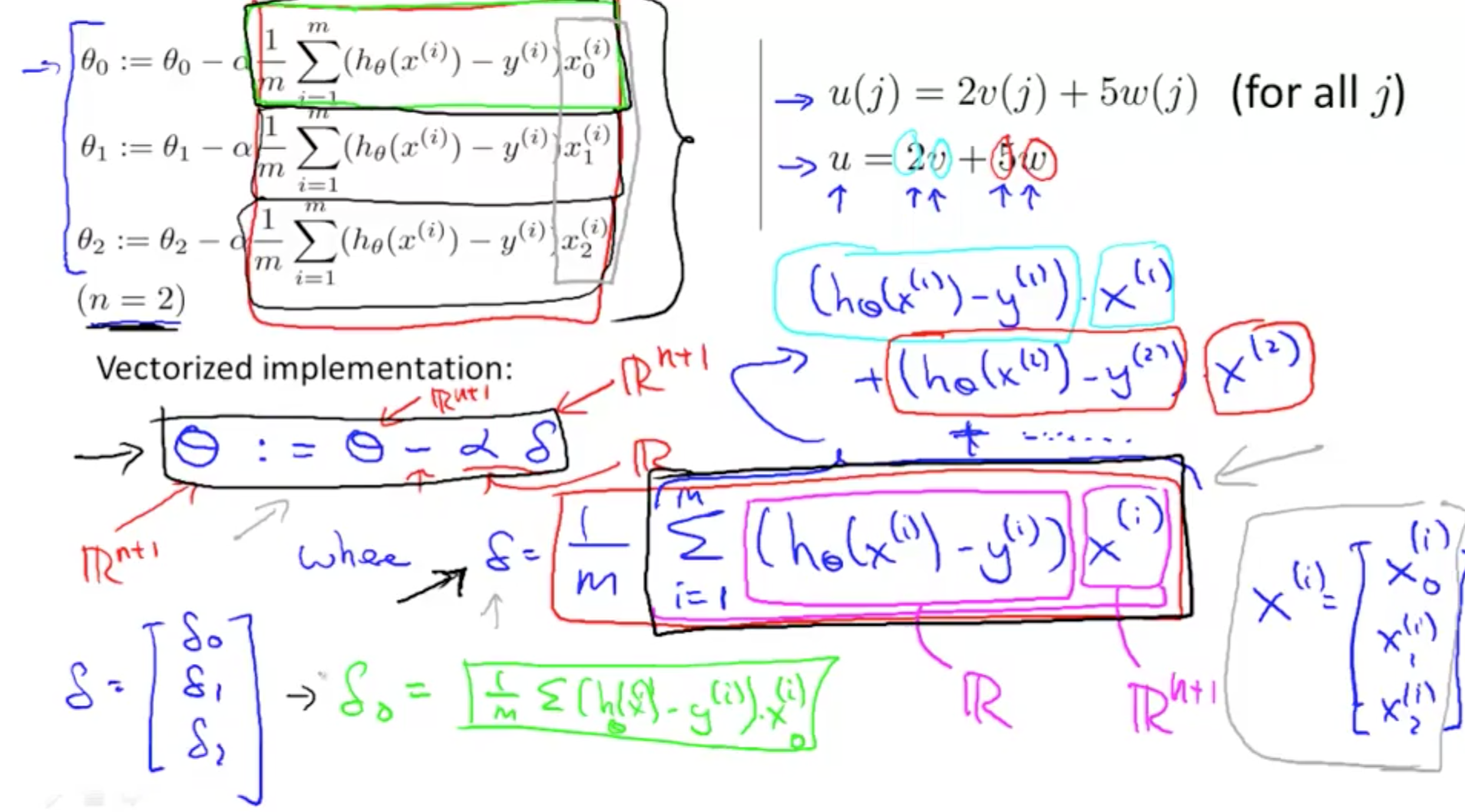This is a short introduction to Octave for Machine Learning.

## 1. Basic Operations

• Not equal to
• 1 ~= 2
• And
• 1 && 2
• Or
• 1 || 2
• Variable
• a = pi
• 3.1316
• Print 2 decimal places
• disp(sprintf('2 decimals: %0.2f', a))
• disp(sprintf('6 decimals: %0.6f', a))
• Longer decimal places
• format short
• a
• Matrices
• 3 x 2
• A = [1 2; 3 4; 5 6]
• Semicolon implies go to the next row
• 1 x 3
• B = [1 2 3]
• 3 x 1
• C = [1; 2; 3]
• 2 x 3 ones
• ones(2, 3)
• D = 2*ones(2,3)
• 1 x 3 zeros
• zeros(1, 3)
• 1 x 3 rand
• rand(1, 3)
• 1 x 3 Gaussian Distribution, mean = 0, SD = 1
• randn(1, 3)
• Start from 1, increment 0.1, up to 2
• v = 1:0.1:2
• Start from 1, increment 1, up to 6
• v = 1:6
• Histogram
• w = -6 + sqrt(10)*(randn(1, 10))
• hist(w)
• 50 bins
• hist(w, 50)
• Identity Matrix
• eye(4)
• 4 x 4
• eye(3)
• 3 x 3
• quit
• press ‘q’

## 2. Moving Data Around

• A = [1 2; 3 4; 5 6]
• Getting matrix dimensions
• size(A)
• get first and second dimension
• size(A, 1)
• size(A, 2)
• Get longest dimension (normally applied to vectors)
• length(A)
• load file.dat
• Show variables in current workspace
• who
• for a more detailed version
• whos
• Get data from file priceY
• v = priceY(1:10)
• Save file
• save hello.txt v -ascii;
• Accessing elements in matrix (array)
• A(3, 2)
• This would give row 6, column 2, so 6
• A(2, :)
• This would give all of row 2
• A(:, 2)
• This would give all of column 2
• A([1,3], :)
• This gives everything from Row 1, 3 and all Columns
• Replace Elements
• A(:, 2) = [10; 11; 12]
• This would replace second column (all rows)
• Append Elements
• A = [A, [100; 101; 102]];
• Appends new column vector [100; 101; 102]
• Put all elements of A into a single vector
• A(:)
• Concatenate Matrices Horizontally
• A = [1 2; 3 4; 5 6]
• B = [4 5; 6 7; 8 9]
• C = [A B]
• Concatenate Matrices Vertically
• C = [A; B]

## 3. Computing on Data

• A = [1 2; 3 4; 5 6]
• B = [11 12; 13 14; 15 16]
• C = [1 1; 2 2]
• Muliply matrices
• A*C
• Element-wise Multiplication
• Literal multiplication of cell to cell
• [1 2] .* [2 2]
• = [2 4]
• Element-wise squaring
• A .^ 2
• Squares each cell
• Element-wise reciprocal
• 1 ./ A
• Element-wise log and exponential
• log(A)
• exp(A)
• Element-wise absolute value
• abs(A)
• Add numbers to each cell
• A + 1
• Transpose (m x n to n x m)
• A'
• Maximum Value of matrix
• max(A)
• Does column-wise maximum
• Comparison
• A < 3
• 1: true
• 0: false
• Finding elements that fulfil inequality (vector)
• find(A<3)
• gives which elements are < 3
• Magic(num)
• Each row, column and diagonal add up to the same thing
• Something like sudoku
• magic(3)
• Find which element in an array fulfills inequality
• [r, c] = find(A >= 7)
•• Sum all elements
• sum(A)
• Product all elements
• prod(A)
• Round down
• floor(A)
• Round up
• ceil(A)
• Create random matrix
• rand(3)
• max(rand(3), rand(3))
• This takes max of 2 3x3 matrices
• Finding max per column
• max(A, [], 1)
• Finding max per row
• max(A, [], 2)
• Finding max of all numbers
• max(max(A))
• Converting to vector
• A(:)
• Sum each column
• sum(A, 1)
• Sum each row
• sum(A, 2)
• Sum diagonal
• A .* eye(9)
• Assuming A is 9 x 9
• sum(sum(A .* eye(9)))
• sum(sum(A .* flipud(eye(9))))
• Inverse Matrix
• pinv(A)

## 4. Plotting Data

• t = [0:0.01:0.98];
• y1 = sin(2*pi*4*t);
• plot(t, y1);
• hold on;
• This ‘hold on’ allows you to print two graphs together
• y2 = cos(2*pi*4*t);
• plot(t, y2);
• xlabel('time')
• ylabel('value')
• legend('sin', 'cos')
• title('my plot')
• rint -dpng 'myPlot.png'
• Save file
• close
• Get rid of figure
• figure(1); plot(t, y1)
• figure(2); plot(t, y2)
• Multiple figures
• subplot(1, 2, 1); 
• plot(t, y1); 
• Divides plot a 1 x 2 grid, access first element
• subplot(1, 2, 2); 
• plot(t, y2); 
• Divides plot a 1 x 2 grid, access second element
• axis([0.5 1 -1 1])
• x-axis: 0.5 to 1
• y-axis: -1 to 1
• clf
• Clear figure
• imagesc(A), colorbar, colormap gray`
• Three commands using comma to chain commands
• Determine concentration of numbers on a grid

• for
• while
• else

## 6. Vectorization

• Matlab/Octave index starts from 1
• Transposing theta would have a more simpler and efficient code
• Implementation in Octave
• Compress for loop to one line of vectorized code• Vectorized implementation of gradient descent
• Delta and Theta are vectors
• Elements of Delta Vector (black boxes)
• Elements of Theta Vector (Theta0, Theta1, Theta2)### Credits

I would like to give full credits to the respective authors as these are my personal python notebooks taken from deep learning courses from Andrew Ng, Data School and Udemy :) This is a simple python notebook hosted generously through Github Pages that is on my main personal notes repository on https://github.com/ritchieng/ritchieng.github.io. They are meant for my personal review but I have open-source my repository of personal notes as a lot of people found it useful.

Tags: